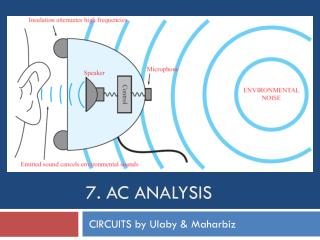DownloadDownload Presentation7. AC Analysis

# 7. AC Analysis

Télécharger la présentation## 7. AC Analysis

- - - - - - - - - - - - - - - - - - - - - - - - - - - E N D - - - - - - - - - - - - - - - - - - - - - - - - - - -
##### Presentation Transcript

1. 7. AC Analysis CIRCUITS by Ulaby & Maharbiz

2. Overview

3. Linear Circuits at ac Objective:Todetermine the steady state response of a linear circuit to ac signals • Sinusoidal input is common in electronic circuits • Any time-varying periodic signal can be represented by a series of sinusoids (Fourier Series) • Time-domain solution method can be cumbersome

4. Sinusoidal Signals Useful relations

6. Complex Numbers We will find it is useful to represent sinusoids as complex numbers Rectangular coordinates Polar coordinates Relations based on Euler’s Identity

7. Relations for Complex Numbers Learn how to perform these with your calculator/computer

8. Phasor Domain 1. The phasor-analysis technique transforms equations from the time domain to the phasor domain. 2. Integro-differential equations get converted into linear equations with no sinusoidal functions. 3. After solving for the desired variable--such as a particular voltage or current-- in the phasor domain, conversion back to the time domain provides the same solution that would have been obtained had the original integro-differential equations been solved entirely in the time domain.

9. Phasor Domain Phasor counterpart of

10. Time and Phasor Domain It is much easier to deal with exponentials in the phasor domain than sinusoidal relations in the time domain. You just need to track magnitude/phase, knowing that everything is at frequency w.

11. Phasor Relation for Resistors Current through a resistor Time domain Time Domain Frequency Domain Phasor Domain

12. Phasor Relation for Inductors Current through inductor in time domain Time domain Phasor Domain Time Domain

13. Phasor Relation for Capacitors Voltage across capacitor in time domain is Time domain Time Domain Phasor Domain

14. Summary of R, L, C

15. ac Phasor Analysis General Procedure Using this procedure, we can apply our techniques from dc analysis

16. Example 1-4: RL Circuit cont.

17. Resistor Inductor Capacitor Impedance and Admittance Impedance is voltage/current Admittance is current/voltage R = resistance = Re(Z) G = conductance = Re(Y) X = reactance = Im(Z) B = susceptance = Im(Y)

18. Impedance Transformation

19. Voltage & Current Division

20. Cont.

21. Example 7-6: Input Impedance (cont.)

22. Example 7-9: Thévenin Circuit

23. Linear Circuit Properties Thévenin/Norton and Source Transformation Also Valid

24. Phasor Diagrams

25. Phase-Shift Circuits

27. Node 1 Cont.

28. (cont.) Cont.

29. (cont.)

30. Example 7-14: Mesh Analysis by Inspection

31. Example 7-16: Thévenin Approach

32. Example 7-16: Thévenin Approach (Cont.)

33. Example 7-16: Thévenin Approach (Cont.)

34. Power Supply Circuit

35. Ideal Transformer

36. Half-Wave Rectifier

37. Full-Wave Rectifier Current flow during first half of cycle Current flow during second half of cycle

38. Smoothing RC Filter

39. Complete Power Supply

40. Example 7-20: Multisim Measurement of Phase Shift

41. Example 7-20 (cont.) Using Transient Analysis

42. Summary